特征点提取_特征点提取算法 - CSDN

• 特征匹配（Feature Match)是计算机视觉中很多应用的基础，所以花一些时间去深入理解这个概念是不为过的。本文希望通过一种通俗易懂的方式来阐述特征匹配这个过程，以及在过程中遇到的一些问题。 概念理解： 假设...
特征匹配（Feature Match)是计算机视觉中很多应用的基础，所以花一些时间去深入理解这个概念是不为过的。本文希望通过一种通俗易懂的方式来阐述特征匹配这个过程，以及在过程中遇到的一些问题。

概念理解：

假设这样的一个场景，小明和小小明都在看一个图片，但是他们想知道他们看的是否是同一幅图片，于是他们就通过电话描述这个图片，来判断是否是同一个图片。比如说有下面两个图片对话1：

小白：我的图片里面有五个很明显的特征，分别在图像的上下左右中五个位置。

小黑：我的图片里面也有五个很明显的特征，分别在图像的上下左右中五个位置。

对话2：

小白：我的一个特征左边是三角形，右边是圆形，上面是菱形，下面是正方形。

小黑：我也有一个特征左边是三角形，右边是圆形，上面是菱形，下面是正方形。

对话3：

小白和小黑：那我们看到的就是同一个特征了。

上述三个对话其实分别代表这特征提取，特征描述和特征匹配。

那么来详细的分析一下，我们的问题是要匹配两张图像是否是同一个图像，比较好的方法就是找出图像中特征显著的内容然后来进行比较，如果这些特征都一致，那么就有很高的概率称他们为同一个图像。所以首先第一步就是找出图像中特征性强的内容（Feature Detect），上图中特征性强的就是五个红色的图案。但是只知道有显著特征没用，必须知道两张图像中的特征是不是一致的，如何判断特征是不是一致的，就需要我们对这个特征进行描述（Feature Descriptor），如果描述非常的相似或者说是相同，那么就可以判断为是同一特征。那么问题来了，该如何去描述一个特征，什么样的描述是一个好的描述呢。从对话2中我们可以看出，描述一个特征其实就是描述特征与他周围内容的相互关系。那么什么样的描述是一个好的描述呢，就要提到我们为什么要描述特征了？我们描述特征是为了能够更好的匹配特征，使得我们认为描述相同的特征是同一个特征的是可信的（概率高的）。所以我们的描述必须是有代表性的，具有排他性的（discriminative）,而不是模棱两可泛泛而谈的。最后我们就可以根据描述的相似性来判断这对特征是否是同一个特征。

特征不变性的理解：

接下来我们将谈一下特征的不变性。

旋转不变性：
再看下面两张图片：于是我们在看审视三个对话，你会发现对话2就改变了。

对话2：

小白：我的一个特征左边是三角形，右边是圆形，上面是菱形，下面是正方形。

小黑：我有一个特征左边是正方形，右边是菱形，上面是三角形，下面是圆形。

于是小黑和小白就认为他们看得并不是同一个图片。但事实上他们看得确实是同一种图片。于是我们就说这种特征不具有旋转不变性。

那么如何实现旋转不变性呢，再看下面两张图片：然后我们再来审视对话2：

对话2：

小白：我的一个特征西边是三角形，东边是圆形，北边是菱形，南边是正方形。

小黑：我也有一个特征西边是三角形，东边是圆形，北边是菱形，南边是正方形。

这时候两个特征的描述就一致了，即拥有了旋转不变性。所以只要对特征定义方向，然后在同一个方向上进行特征描述就可以实现旋转不变性。

尺度不变性
接下来谈一下尺度不变性，依然看下面两张图片：对话2：

小白：我的一个特征东边5个像素的地方有个圆形。

小黑：我也有一个特征东边7个像素的地方有个圆形。

这就是尺度变化造成的特征不匹配，为了实现尺度不变性，就需要给特征加上尺度因子，比如说小白看到的是尺度为5的，小黑看到的是尺度为7的，那么在进行描述的时候，将尺度统一就可以实现尺度不变性了。

所谓的旋转不变性和尺度不变性的原理，就是我们在描述一个特征之前，将两张图像都变换到同一个方向和同一个尺度上，然后再在这个统一标准上来描述这个特征。同样的，如果在描述一个特征之前，将图像变换到同一个仿射尺度或者投影尺度上，那么就可以实现仿射不变性和投影不变性。

总结：

特征匹配的方法是先找出特征显著的特征点（Feature Detect），然后再分别描述两个特征点（Feature Descriptor），最后比较两个描述的的相似程度来判断是否为同一个特征（Feature Match）。而在特征描述之前如果能够做到确定特征的方向，则可以实现旋转不变性（Rotation invarient），如果能确定尺度，则可以实现尺度不变性（Scale invarient）。

转自https://blog.csdn.net/before1993/article/details/51197952
展开全文• 对常用特征点记录总结 图像处理特征不变算子系列之SUSAN算子（三） Susan SUSA（Smallest Univalue Segment Assimilating Nucleus）算子是一种高效的边缘和角点检测算子，并且具有结构保留的降噪功能 原理 ...
参考

对常用特征点记录总结
图像处理特征不变算子系列之Harris算子（二）
图像处理特征不变算子系列之SUSAN算子（三）
【计算机视觉】SIFT中LoG和DoG比较
SIFT特征提取分析
RANSAC算法详解
Harris角点检测原理详解

Susan

SUSA（Smallest Univalue Segment Assimilating Nucleus）算子是一种高效的边缘和角点检测算子，并且具有结构保留的降噪功能

原理

用一个圆形模板在图像上移动，若模板内的像素灰度与模板中心的像素（被称为核Nucleus）灰度值小于一定的阈值，则认为该点与核Nucleus具有相同的灰度，满足该条件的像素组成的区域就称为USAN（Univalue Segment Assimilating Nucleus）。边缘处的点的USAN值小于或等于最大值一半。由此，我们可以得出SUSAN提取边缘和角点算法的基本原理：在边缘或角点处的USAN值最小，可以根据USAN区域的大小来检测边缘、角点等特征的位置和方向信息。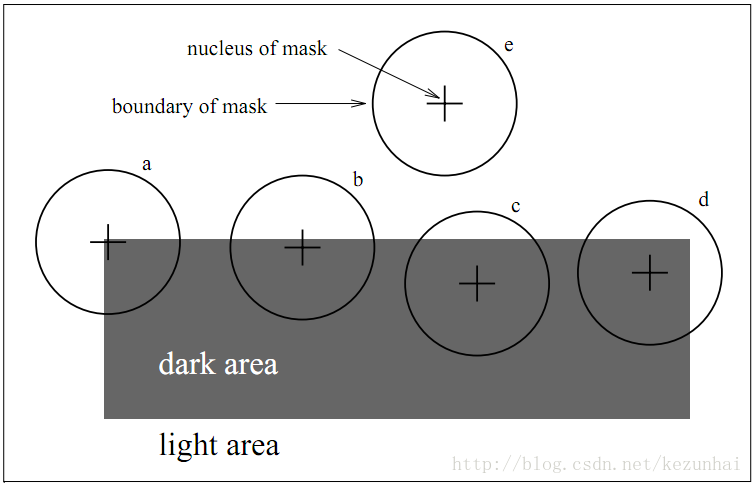实现步骤

利用圆形模板遍历图像，计算每点处的USAN值
设置一阈值g，一般取值为1/2(Max(n)）， 也即取值为USAN最大值的一半，进行阈值化，得到角点响应
使用非极大值抑制来寻找角点

优缺点

完全不涉及梯度的运算，因此其抗噪声能力很强，运算量也比较小
SUSAN算子还是一个各向同性的算子
图像的对比度较大，则可选取较大的t值，而图像的对比度较小，则可选取较小的t值
不仅具有很好的边缘检测性能；而且对角点检测也具有很好的效果。

FAST

FAST( Features from Accelerated Segment Test)由Rosten等人在SUSAN角点特征检测方法的基础上利用机器学习方法提出.

原理

分割测试

在FAST角点检测算子中，一般是通过半径为3.4 pixel、外围16个像素的圆的作为模板筛选特征点。12点分割角点检测算法是在一个图像块上进行。其中p是中心像素点，12点取的是图上用弧线连接的12个点的像素值（通过测试，12点的角点检测性能最稳定、速度更快、效果也很好，有些文献指出9点的方式也很好）。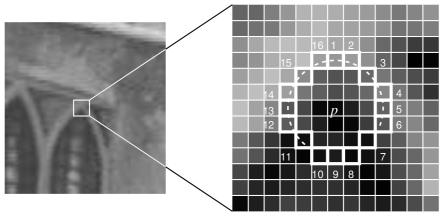t是一个阈值（默认取值为10，不同场景取值有差异），Ip${I}_{p}$$I_p$表示的是中心像素的像素值，Ip→x${I}_{p\to x}$$I_{p{\rightarrow}x}$表示的是圆形模板中的像素值。统计圆形区域中d或b的个数，只要d或b出现的次数大于n（（当是12点分割测试角点检测时，n=12；当是9点时，则n=9），那么该点就被认为是候选角点。

Sp→x=⎧⎩⎨⎪⎪d,Ip→x<Ip−t,s,Ip−t<Ip→x<Ip+t,b,Ip→x>Ip+t,darksimiliarbrighter${S}_{p\to x}=\left\{\begin{array}{ll}d,{I}_{p\to x}<{I}_{p}-t,& \text{dark}\\ s,{I}_{p}-t<{I}_{p\to x}<{I}_{p}+t,& \text{similiar}\\ b,{I}_{p\to x}>{I}_{p}+t,& \text{brighter}\end{array}$
S_{p{\rightarrow}x} =
\begin{cases}
d,  I_{p{\rightarrow}x}<I_p-t,& \text {dark} \\
s, I_p-t < I_{p{\rightarrow}x}<I_p+t,& \text {similiar} \\
b, I_{p{\rightarrow}x}>I_p+t,& \text {brighter}
\end{cases}

在分割测试步骤中，为了加快速度，其实不需要对这些像素进行逐一的比较。简单来说：首先比较1、5、9、13处点的像素值（也即水平方向和垂直方向上的4个点）与中心像素值的大小，如果这四个点中的像素值有3个或3个以上大于Ip→x+t${I}_{p\to x}+t$$I_{p{\rightarrow}x}+t$或小于Ip→x−t${I}_{p\to x}-t$$I_{p{\rightarrow}x}-t$，那么则认为该处是一个候选角点，否则就不可能是角点。

ID3训练

将模板内的像素分成三部分d、s、b，分别记为：Pd${P}_{d}$$P_d$，Ps${P}_{s}$$P_s$，Pb${P}_{b}$$P_b$。因此对于每个Sp→x${S}_{p\to x}$$S_{p{\rightarrow}x}$都属于Pd${P}_{d}$$P_d$，Ps${P}_{s}$$P_s$，Pb${P}_{b}$$P_b$中的一个。另外，令Kp${K}_{p}$$K_p$为true，如果p为角点，否则为false。通过ID3算法来选择具有最大信息增益的像素来判断一个像素是否为角点。Kp的熵用下式来计算：
H(P)=(c+c−)log2(c+c−)−clog2c−c−log2c−$H\left(P\right)=\left(c+{c}^{-}\right){\mathrm{log}}_{2}\left(c+{c}^{-}\right)-c{\mathrm{log}}_{2}c-{c}^{-}{\mathrm{log}}_{2}{c}^{-}$
H(P)=(c+c^-)\log_2(c+c^-)-c{\log_2}c-c^-{\log_2}c^-

上式中c$c$$c$表示角点数量，c−${c}^{-}$$c^-$表示非角点数量。某一像素的信息增益通过下式表示：
H(P)−H(Pd)−H(Ps)−H(Pb)$H\left(P\right)-H\left({P}_{d}\right)-H\left({P}_{s}\right)-H\left({P}_{b}\right)$H(P)-H(P_d)-H(P_s)-H(P_b)

非极大值抑制

在上面的分割测试中，没有计算角点响应函数（Corner Response Function），非极大值抑制无法直接应用于提取的特征。因此，定义一个角点响应函数V，考虑到分割测试的特征以及计算速度的需要，角点响应函数的定义如下：

V=max⎛⎝∑xϵSbright|Ip→x−Ip→|−t,∑xϵSdark|Ip→x−Ip→|−t⎞⎠$V=max\left(\sum _{xϵ{S}_{bright}}|{I}_{p\to x}-{I}_{p\to }|-t,\sum _{xϵ{S}_{dark}}|{I}_{p\to x}-{I}_{p\to }|-t\right)$
V=max
\left(\sum_{x{\epsilon}S_{bright}}| I_{p{\rightarrow}x}- I_{p{\rightarrow}}|-t,\sum_{x{\epsilon}S_{dark}}| I_{p{\rightarrow}x}- I_{p{\rightarrow}}|-t
\right)

实现步骤

对固定半径圆上的像素进行分割测试，通过逻辑测试可以去处大量的非特征候选点；
基于分类的角点特征检测，利用ID3 分类器根据16个特征判决候选点是否为角点特征，每个特征的状态为一1，0，1。
利用非极大值抑制进行角点特征的验证。

优缺点

计算速度快，可以应用于实时场景中
容易受到噪声影响，阈值t的影响也较大

BRIEF

Brief为特征描述子，对已检测到的特征点进行描述，是一种二进制编码描述子

实现步骤

为减少噪声干扰，先对图像进行高斯滤波（方差2，窗口9x9）
以特征点为中心，取SxS的邻域大窗口。在大窗口中随机选取(有5种经典方法)一对（两个）5x5的子窗口，比较子窗口内的像素和（可用积分图像完成），进行二进制赋值。（一般S=31），其中p(x)$p\left(x\right)$$p(x)$,p(y)$p\left(y\right)$$p(y)$分别为所在5x5子窗口的像素和
τ(p;x,y):={1,0,if p(x)<p(y)otherwise\tau(p;x,y):=\begin{cases} 1,& \text {if $p(x)$<$p(y)$} \\
0,& \text {otherwise}\end{cases}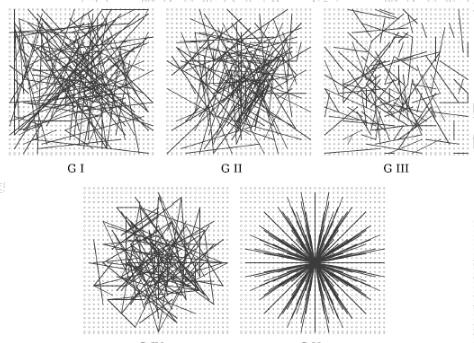在大窗口中随机选取N对子窗口，重复步骤2的二进制赋值，形成一个二进制编码，这个编码就是对特征点的描述，即特征描述子。（一般N=256）构造一个512个bit的BRIEF，就需要512对[x,y]，且需要注意，它们是有序的，每次计算位置都相同，否则影响最终结果。也就说说，一旦选定了512对[x,y]，那么，无论是提取特征，还是匹配特征，都要按照这512对进行计算。
用汉明距离进行配对。两个特征编码对应bit位上相同元素的个数小于128的，一定不是配对的。一幅图上特征点与另一幅图上特征编码对应bit位上相同元素的个数最多的特征点配成一对。

优缺点

抛弃了传统的用梯度直方图描述区域的方法，改用检测随机响应，大大加快了描述子建立速度
生成的二进制描述子便于高速匹配，计算Hamming距离只需通过异或操作加上统计二进制编码中“1”的个数的操作，这些通过底层的运算即可实现
缺点很明显就是旋转不变性较差

ORB

DoG

DoG算子是由Lowe D.G.提出的，对噪声、尺度、仿射变化和旋转等具有很强的鲁棒性，能够提供更丰富的局部特征信息

原理

尺度空间

在用机器视觉系统分析未知场景时，机器并不知道图像中物体的尺度，只有通过对图像的多尺度描述，才能获得对物体感知的最佳尺度。如果在不同尺度上，对输入的图像都能检测到相同的关键点特征，那么在不同尺度下也可以实现关键点的匹配，从而实现关键点的尺度不变特性。

图像金字塔多分辨率

金字塔是早期图像多尺度的表示形式，图像金字塔一般包括2个步骤，分别是使用低通滤波平滑图像；对图像进行降采样（也即图像缩小为原来的1/4，长宽高缩小为1/2），从而得到一系列尺寸缩小的图像。金字塔的构造如下所示：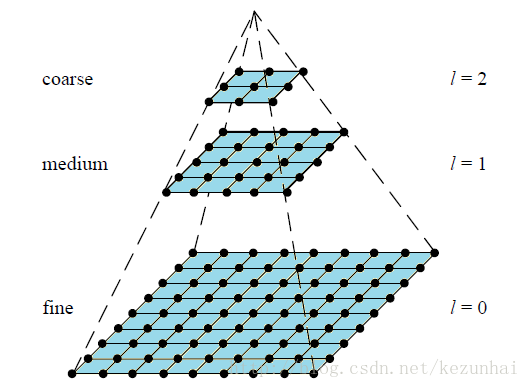高斯平滑滤波

高斯核是唯一可以产生多尺度空间的核。一个图像的尺度空间L(x,y,σ) ,定义为原始图像I(x,y)与一个可变尺度的2维高斯函数G(x,y,σ)卷积运算。 二维空间高斯函数：
G(xi,yi,σ)=12πσ2exp(−(x−xi)2+(y−yi)22σ2)$G\left({x}_{i},{y}_{i},\sigma \right)=\frac{1}{2\pi {\sigma }^{2}}exp\left(-\frac{\left(x-{x}_{i}{\right)}^{2}+\left(y-{y}_{i}{\right)}^{2}}{2{\sigma }^{2}}\right)$G(x_i,y_i,\sigma)=\frac{1}{2\pi{{\sigma}^2}}exp(-\frac{(x-x_i)^2+(y-y_i)^2}{2{\sigma}^2})
尺度空间表示为：
L(x,y,σ)=G(x,y,σ)∗I(x,y)$L\left(x,y,\sigma \right)=G\left(x,y,\sigma \right)\ast I\left(x,y\right)$L(x,y,\sigma)=G(x,y,\sigma)*I(x,y)
高斯模版是圆对称的，且卷积的结果使原始像素值有最大的权重，距离中心越远的相邻像素值权重也越小。高斯模糊另一个重要的性质就是线性可分，使用二维矩阵变换的高斯模糊可以通过在水平和竖直方向各进行一维高斯矩阵变换相加得到。

多尺度与多分辨率

尺度空间表达和金字塔多分辨率表达之间最大的不同是：
1. 尺度空间表达是由不同高斯核平滑卷积得到，在所有尺度上有相同的分辨率；
2. 金字塔多分辨率表达每层分辨率减少固定比率。
所以，金字塔多分辨率生成较快，且占用存储空间少；而多尺度表达随着尺度参数的增加冗余信息也变多。多尺度表达的优点在于图像的局部特征可以用简单的形式在不同尺度上描述；而金字塔表达没有理论基础，难以分析图像局部特征。

拉普拉斯金字塔

结合尺度空间表达和金字塔多分辨率表达，就是在使用尺度空间时使用金字塔表示，在计算机视觉中最有名莫过于拉普拉斯金字塔。拉普拉斯金字塔顾名思义就是通过对图像进行拉普拉斯操作，然后进行一个降采样的过程。具体来说就是：原始图像作为金字塔的底层，也即0层，称为g0${g}_{0}$$g_0$，对0层图像g0${g}_{0}$$g_0$进行进行拉普拉斯金操作，得到第一层图像g1${g}_{1}$$g_1$；接着对第一层图像进行拉普拉斯操作，得到第二层图像g2${g}_{2}$$g_2$，依次类推，并进行一个降采样，如此构造拉普拉斯金字塔。

DoG金字塔

DoG（Difference of Gaussian）其实是对高斯拉普拉斯(LoG)的近似，在某一尺度上的特征检测可以通过对两个相邻高斯尺度空间的图像相减，得到DoG的响应值图像D(x,y,σ)$D\left(x,y,\sigma \right)$$D(x,y,\sigma)$，这比直接计算LoG效率更高。

对原图进行相邻尺度的高斯滤波
g1=Gσ1(x,y)∗f(x,y)${g}_{1}={G}_{{\sigma }_{1}}\left(x,y\right)\ast f\left(x,y\right)$g_1=G_{{\sigma}_1}(x,y)*f(x,y)
g2=Gσ2(x,y)∗f(x,y)${g}_{2}={G}_{{\sigma }_{2}}\left(x,y\right)\ast f\left(x,y\right)$g_2=G_{{\sigma}_2}(x,y)*f(x,y)
将上面的滤波得到的两幅图像相减：
g1(x,y)−g2(x,y)=Gσ1∗f(x,y)−Gσ2∗f(x,y)=(Gσ1−Gσ2)∗f(x,y)=DoG∗f(x,y)${g}_{1}\left(x,y\right)-{g}_{2}\left(x,y\right)={G}_{{\sigma }_{1}}\ast f\left(x,y\right)-{G}_{{\sigma }_{2}}\ast f\left(x,y\right)=\left({G}_{{\sigma }_{1}}-{G}_{{\sigma }_{2}}\right)\ast f\left(x,y\right)=DoG\ast f\left(x,y\right)$g_1(x,y)-g_2(x,y)=G_{{\sigma}_1}*f(x,y)-G_{{\sigma}_2}*f(x,y)=(G_{{\sigma}_1}-G_{{\sigma}_2})*f(x,y)=DoG*f(x,y)
将公式简化写法：
D(x,y,σ)=(G(x,y,kσ)−G(x,y,σ))⨂I(x,y)$D\left(x,y,\sigma \right)=\left(G\left(x,y,k\sigma \right)-G\left(x,y,\sigma \right)\right)⨂I\left(x,y\right)$D(x,y,\sigma)=(G(x,y,k\sigma)-G(x,y,\sigma)){\bigotimes}I(x,y)=L(x,y,kσ)−L(x,y,σ)$=L\left(x,y,k\sigma \right)-L\left(x,y,\sigma \right)$=L(x,y,k\sigma)-L(x,y,\sigma)
构造高斯金字塔
为了得到DoG图像，首先要构造高斯金字塔，高斯金字塔在多分辨率金字塔的基础上加入了高斯滤波，也就是对金字塔每层图像采用不同的参数sigma进行了高斯卷积，使得金字塔的每层有多张图片组成为一个Octave，每组有多张（也叫层interval）图像。每个Octave是由同一大小的图像，经过不同sigma高斯滤波得到的，而Interval则表示的是同一个sigma高斯滤波的图像。另外，降采样时，金字塔上边一组图像的第一张图像（最底层的一张）是由前一组（金字塔下面一组）图像的倒数第三张隔点采样得到，图像表示如下：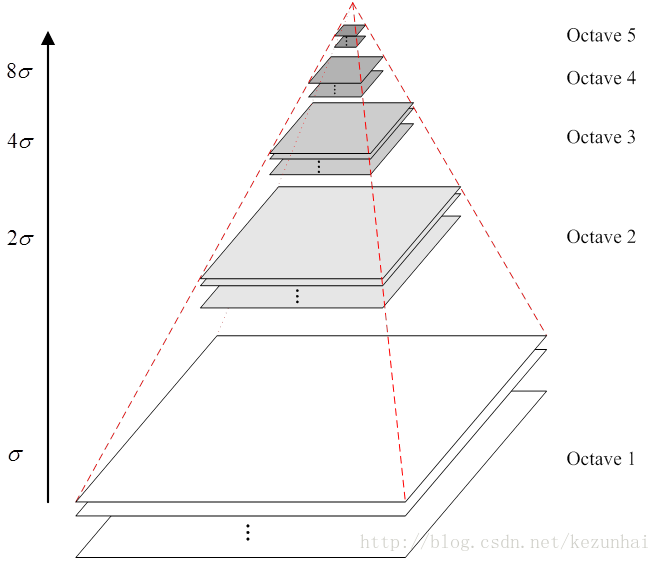求DoG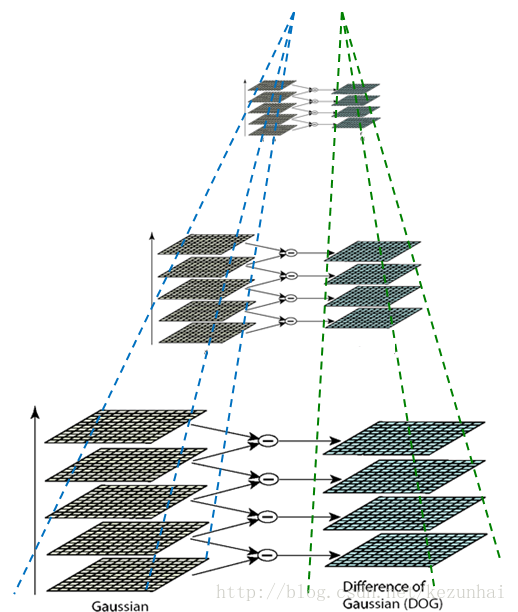求角点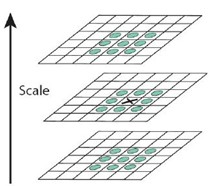X标记当前像素点，绿圈标记邻接像素点，用这个方式，最多检测相邻尺度的26个像素点。如果它是所有邻接像素点的最大值或最小值点，则X被标记为特征点，如此依次进行，则可以完成图像的特征点提取。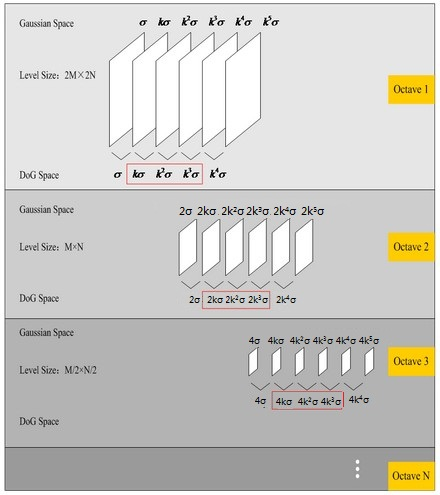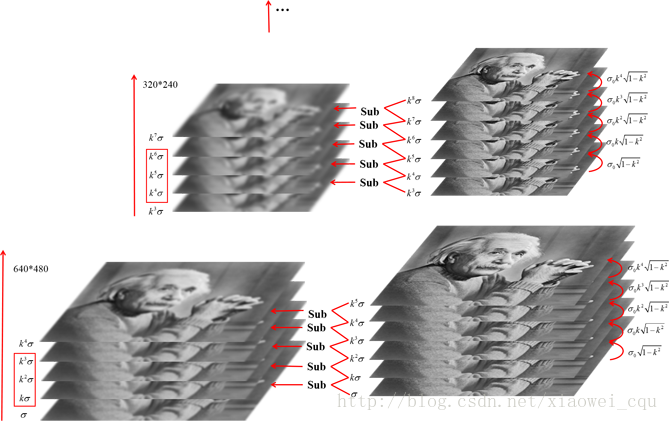优缺点

具有尺度不变特性，抗旋转
计算量大

RANSC特征点筛选

随机抽样一致算法（random sample consensus,RANSAC）,采用迭代的方式从一组包含离群的被观测数据中估算出数学模型的参数。

RANSAC算法的基本假设是样本中包含正确数据(inliers，可以被模型描述的数据)，也包含异常数据(outliers，偏离正常范围很远、无法适应数学模型的数据)，即数据集中含有噪声。这些异常数据可能是由于错误的测量、错误的假设、错误的计算等产生的。同时RANSAC也假设，给定一组正确的数据，存在可以计算出符合这些数据的模型参数的方法。

实现步骤

假定模型（如直线方程），并随机抽取Nums个（以2个为例）样本点，对模型进行拟合
由于不是严格线性，数据点都有一定波动，假设容差范围为：sigma，找出距离拟合曲线容差范围内的点，并统计点的个数
重新随机选取Nums个点，重复第一步~第二步的操作，直到结束迭代
每一次拟合后，容差范围内都有对应的数据点数，找出数据点个数最多的情况，就是最终的拟合结果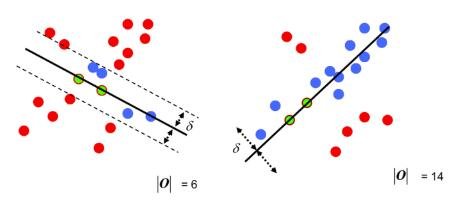优缺点

最小二乘法适用于有效数据占大多数，无效数据少的情况，是从一个整体误差最小的角度去考虑。RANSC基于假设寻找最优。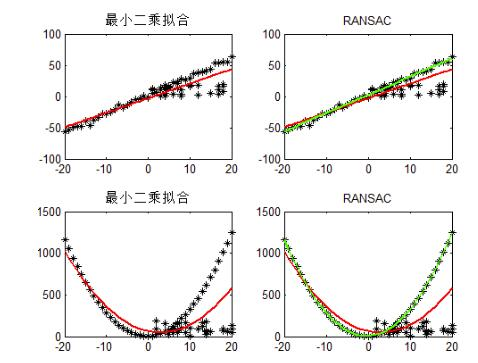Harris角点检测

原理

用一个固定窗口在图像上进行任意方向上的滑动，比较滑动前与滑动后两种情况，窗口中的像素灰度变化程度，如果存在任意方向上的滑动，都有着较大灰度变化，那么我们可以认为该窗口中存在角点。

数学刻画
E(U,V)=∑x,yw(x,y)[I(x+u,y+v)−I(x,y)]2$E\left(U,V\right)=\sum _{x,y}w\left(x,y\right)\left[I\left(x+u,y+v\right)-I\left(x,y\right){\right]}^{2}$E(U,V)=\sum_{x,y}w(x,y)[I(x+u,y+v)-I(x,y)]^2
式中，[u,v]$\left[u,v\right]$$[u,v]$是窗口的偏移量，w(x,y)$w\left(x,y\right)$$w(x,y)$是窗口的权重系数。一般可用高斯二维函数。
表达式演化-泰勒展开
f(x+u,y+v)≈f(x,y)+ufx(x,y)+vfy(x,y)$f\left(x+u,y+v\right)\approx f\left(x,y\right)+u{f}_{x}\left(x,y\right)+v{f}_{y}\left(x,y\right)$f(x+u,y+v){\approx}f(x,y)+uf_x(x,y)+vf_y(x,y)

∑[I(x+u,y+v)−I(x,y)]2≈∑[I(x,y)+uIx+vIy−I(x,y)]2=∑u2Ix2+2uvIxIy+v2Iy2=∑[uv][Ix2IxIyIxIyIy2][uv]=[uv](∑[Ix2IxIyIxIyIy2])[uv](1)(2)(3)(4)$\begin{array}{}\text{(1)}& \sum \left[I\left(x+u,y+v\right)-I\left(x,y\right){\right]}^{2}& \approx \sum \left[I\left(x,y\right)+u{I}_{x}+v{I}_{y}-I\left(x,y\right){\right]}^{2}\text{(2)}& & =\sum {u}^{2}{{I}_{x}}^{2}+2uv{I}_{x}{I}_{y}+{v}^{2}{{I}_{y}}^{2}\text{(3)}& & =\sum \left[\begin{array}{cc}u& v\end{array}\right]\left[\begin{array}{cc}{{I}_{x}}^{2}& {I}_{x}{I}_{y}\\ {I}_{x}{I}_{y}& {{I}_{y}}^{2}\end{array}\right]\left[\begin{array}{c}u\\ v\end{array}\right]\text{(4)}& & =\left[\begin{array}{cc}u& v\end{array}\right]\left(\sum \left[\begin{array}{cc}{{I}_{x}}^{2}& {I}_{x}{I}_{y}\\ {I}_{x}{I}_{y}& {{I}_{y}}^{2}\end{array}\right]\right)\left[\begin{array}{c}u\\ v\end{array}\right]\end{array}$\begin{align}
{\sum}[I(x+u,y+v)-I(x,y)]^2 & {\approx}{\sum}[I(x,y)+uI_x+vI_y-I(x,y)]^2 \\
& =\sum u^2{I_x}^2+2uvI_xI_y+v^2{I_y}^2 \\
& = \sum\begin{bmatrix} u & v \\  \end{bmatrix} \begin{bmatrix} {I_x}^2 & I_xI_y \\   I_xI_y & {I_y}^2 \end{bmatrix} \begin{bmatrix} u\\ v  \\  \end{bmatrix}\\
& = \begin{bmatrix} u & v \\  \end{bmatrix} ({\sum}\begin{bmatrix} {I_x}^2 & I_xI_y \\   I_xI_y & {I_y}^2 \end{bmatrix}) \begin{bmatrix} u\\ v  \\  \end{bmatrix}\\
\end{align}

E(u,v)可更新为$E\left(u,v\right)可更新为$$E(u,v)可更新为$
E(u,v)≈[uv]M[uv]$E\left(u,v\right)\approx \left[\begin{array}{cc}u& v\end{array}\right]M\left[\begin{array}{c}u\\ v\end{array}\right]$E(u,v)\approx\begin{bmatrix} u & v \\  \end{bmatrix} M\begin{bmatrix} u\\ v  \\  \end{bmatrix}
M=∑x,yw(x,y)[Ix2IxIyIxIyIy2]$M=\sum _{x,y}w\left(x,y\right)\left[\begin{array}{cc}{{I}_{x}}^{2}& {I}_{x}{I}_{y}\\ {I}_{x}{I}_{y}& {{I}_{y}}^{2}\end{array}\right]$M=\sum_{x,y}w(x,y) \begin{bmatrix} {I_x}^2 & I_xI_y \\   I_xI_y & {I_y}^2 \end{bmatrix}

实现步骤

对每一个像素计算自相关矩阵M
构造角点性映射图
M=[ACCB]$M=\left[\begin{array}{cc}A& C\\ C& B\end{array}\right]$M= \begin{bmatrix}A &C \\   C & B \end{bmatrix}
C(x,y)=det(M)−k(trace(M))2$C\left(x,y\right)=det\left(M\right)-k\left(trace\left(M\right){\right)}^{2}$C(x,y)=det(M)-k(trace(M))^2
det(M)=λ1λ2=AB−C2$det\left(M\right)={\lambda }_{1}{\lambda }_{2}=AB-{C}^{2}$det(M)={\lambda}_1{\lambda}_2=AB-C^2
trace(M)=λ1+λ2=A+B$trace\left(M\right)={\lambda }_{1}+{\lambda }_{2}=A+B$trace(M)={\lambda}_1+{\lambda}_2=A+B
阈值化，对得到的C(x,y)进行阈值分割$C\left(x,y\right)进行阈值分割$$C(x,y)进行阈值分割$
非极大值抑制

优缺点

针对Moravec算子的不足进行了改进，提高了特征点的检测率
Harris算子计算量大，对尺度很敏感，不具有尺度不变性
Harris对特征点的定位也不是很精确
Harris也是各向异性的，对噪声敏感。


展开全文• ## 特征点提取

千次阅读 2018-08-07 15:44:41
作用：特征点提取在“目标识别、图像拼接、运动跟踪、图像检索、自动定位”等研究中起着重要作用； 主要算法： •FAST ,Machine Learning forHigh-speed Corner Detection, 2006 •SIFT,Distinct...
知乎链接

特征提取算法（多种角度比较sift/surf/brisk/orb/akze/freak/gftt）

一、基本概念：

作用：特征点提取在“目标识别、图像拼接、运动跟踪、图像检索、自动定位”等研究中起着重要作用；

主要算法：

•FAST ,Machine Learning forHigh-speed Corner Detection, 2006

•SIFT,Distinctive ImageFeatures from Scale-Invariant Keypoints,2004,invariant
to image translation, scaling, and rotation, partially invariant
toillumination changes and robust to local geometric distortion

•SURF,Speeded Up RobustFeatures,2006,受SIFT启发，比SIFT快，健壮

•ORB,ORB: an efficientalternative to SIFT or SURF,2011，基于FAST，比SIFT快两个数量级，可作为SIFT的替代

•BRISK，BRISK:Binary Robust Invariant Scalable Keypoints

•STAR，Censure: Centersurround extremas for realtime featuredetection and matching,引用次数不高

•MSER，RobustWide Baseline Stereo from Maximally Stable ExtremalRegions,2002,斑点检测

•GFTT，GoodFeatures to Track,1994,Determines strong corners on animage

•HARRIS,Harris and M. Stephens (1988).“A combined corner and edge detector”,也是一种角点检测方法

•FREAK

•AKAZE等

其中标红的5项是在OpenCV中已经进行了实现的。

OpenCV 中和 2D 特征检测相关的算法的头文件位于

opencv\modules\features2d\include\opencv2\features2d.hpp，除SIFT、SURF以外的特征。
opencv\modules\nonfree\include\opencv2\nonfree\features2d.hpp，只包含SIFT、SURF这两个受专利保护的特征，因此不是免费的（nonfree）。

我们来看具体的类
DescriptorExtractor 的子类都是描述子提取器，包含

FREAK
OpponentColorDescriptorExtractor
BriefDescriptorExtractor

FeatureDetector 的子类都是特征检测器，包含

MSER
StarDetector，又名 StarFeatureDetector
FastFeatureDetector
GFTTDetector，又名 GoodFeaturesToTrackDetector
SimpleBlobDetector
DenseFeatureDetector

FeatureDetector 还提供一系列特殊的适配器子类，用于增强或加速之前的 FeatureDetector 类

Feature2D 的子类既是 FeatureDetector，又是 DescriptorExtractor，包含

BRISK
ORB
SIFT
SURF
opencv3.2 contrib模块还加入了深度特征VGG

特征点识别主要流程为:

1、检测关键点、提取描述向量和特征匹配；

2、通过检测关键点和提取描述向量构造出局部特征描述子，

3、然后进行特征匹配

提纯：ransac，ground trurh，NCC，SSDA

BeMatcher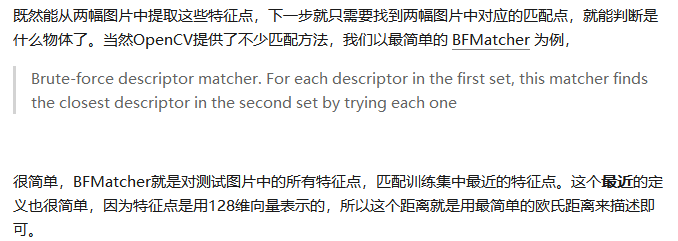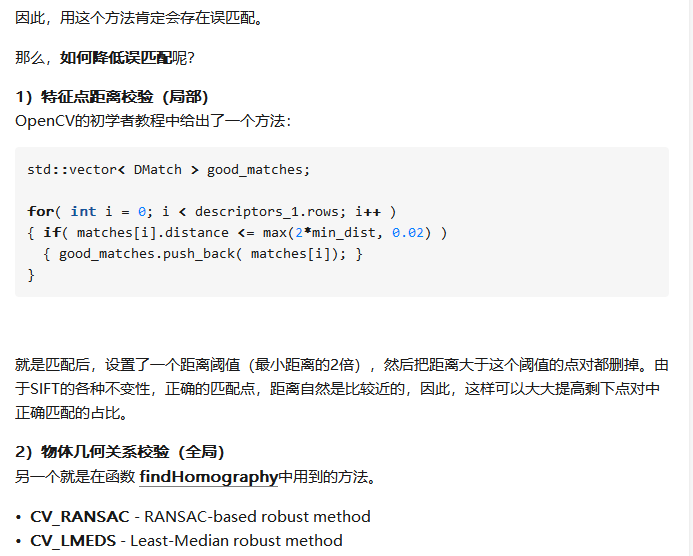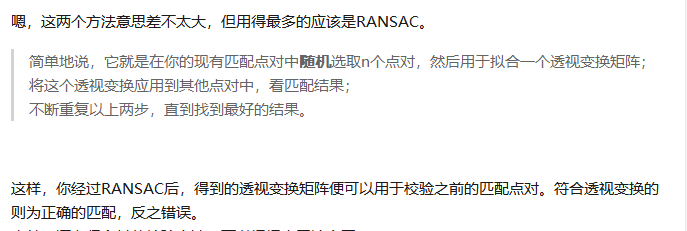二、数据准备：

数据集为pascal中取出的6个数据，分别针对特征点提取的6个方面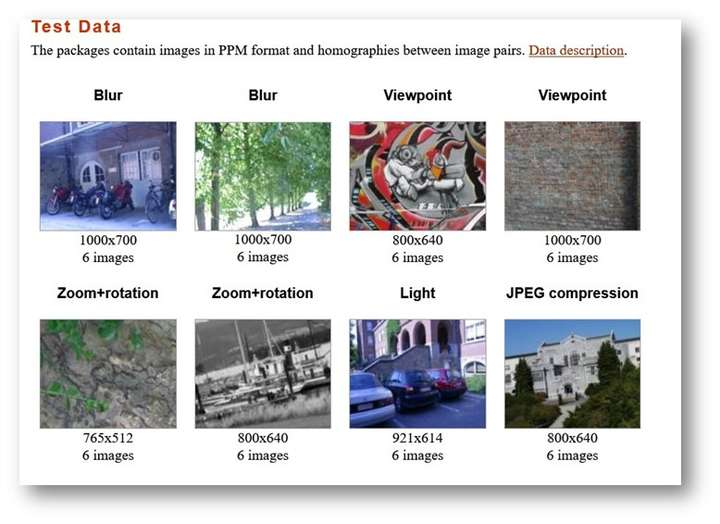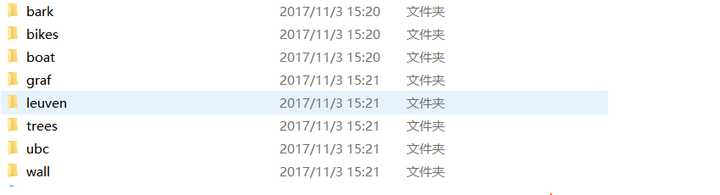其中特征点识别在以下6个方面进行比较

1、算法匹配速度比较 （ubc）

测试方法：在相同的匹配环境下，即使用同样配置的计算机，对相同的一对图像进行比较，测试算法的执行时间

2、旋转变换鲁棒性比较 （bark）

测试方法：对同一图像进行一定角度的旋转，旋转角度逐步递增，旋转后的图像逐一与原始图像进行匹配，比较能够正确匹配的特征点对数，并观察正确匹配对数的变化幅度

3、模糊变换鲁棒性比较 （bikes）

测试方法：对同一图像用不同的高斯核进行模糊处理，模糊处理后的图像逐一与原始图像进

行匹配，比较能够正确匹配的特征点对数，并观察正确匹配对数的变化幅度

4、光照变换鲁棒性比较 （leuven）

测试方法：对同一图像的亮度进行改变，逐

渐降低亮度，改变亮度后的图像逐一与原始图像进行匹配，比较能够正确匹配的特征点对数，并观察正确匹配对数的变化幅度

5、尺度变换鲁棒性比较 （bark）

测试方法：对原图像的尺度大小进行改变，尺度变化后的图像逐一与原始图像进行匹配，比较能够正确匹配的特征点对数，并观察正确匹配对数的变化幅度

6、视角变换鲁棒性比较 （graf）

测试方法：对原场景转一定角度进行拍摄，不同视角的图像逐一与原始图像进行匹配，比较能够正确匹配的特征点对数，并观察正确匹配对

数的变化幅度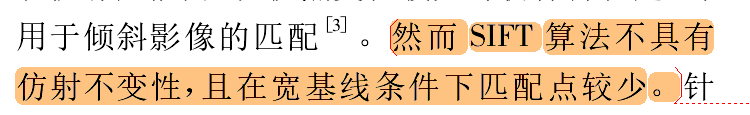展开全文• ## SIFT特征点提取

万次阅读 多人点赞 2018-01-15 18:08:45
计算机视觉中的特征点提取算法比较多，但SIFT除了计算比较耗时以外，其他方面的优点让其成为特征点提取算法中的一颗璀璨的明珠。SIFT算法的介绍网上有很多比较好的博客和文章，我在学习这个算法的过程中也参看网上...
      计算机视觉中的特征点提取算法比较多，但SIFT除了计算比较耗时以外，其他方面的优点让其成为特征点提取算法中的一颗璀璨的明珠。SIFT算法的介绍网上有很多比较好的博客和文章，我在学习这个算法的过程中也参看网上好些资料，即使评价比较高的文章，作者在文章中对有些比较重要的细节、公式来历没有提及，可能写博客的人自己明白，也觉得简单，因此就忽略了这些问题，但是对刚入门的人来说，看这些东西，想搞清楚这些是怎么来的还是比较费时费力的。比如SIFT算法中一个重要的操作：求取描述子的主方向。好多文章只是一提而过或忽略，然后直接给出一个公式，SIFT算法的原作者也提使用抛物线插值，但是具体怎么插的就不太详尽了，对于初学者来说更是不知所云。因此本文打算在参看的文章上对有关这些细节给出一些比较详细的说明，还有本文尽量对操作过程配备对应图片或示意图说明，同时附上robwhesss开源SIFT
C代码对应程序块并给予注解，方便理解。

一、 SIFT算法
1、算法简介
尺度不变特征转换即SIFT (Scale-invariant feature transform)是一种计算机视觉的算法。它用来侦测与描述影像中的局部性特征，它在空间尺度中寻找极值点，并提取出其位置、尺度、旋转不变量，此算法由 David Lowe在1999年所发表，2004年完善总结。
其应用范围包含物体辨识、机器人地图感知与导航、影像缝合、3D模型建立、手势辨识、影像追踪和动作比对。
局部影像特征的描述与侦测可以帮助辨识物体，SIFT特征是基于物体上的一些局部外观的兴趣点而与影像的大小和旋转无关。对于光线、噪声、些微视角改变的容忍度也相当高。基于这些特性，它们是高度显著而且相对容易撷取，在母数庞大的特征数据库中，很容易辨识物体而且鲜有误认。使用 SIFT特征描述对于部分物体遮蔽的侦测率也相当高，甚至只需要3个以上的SIFT物体特征就足以计算出位置与方位。在现今的电脑硬件速度下和小型的特征数据库条件下，辨识速度可接近即时运算。SIFT特征的信息量大，适合在海量数据库中快速准确匹配。
SIFT算法的实质是在不同的尺度空间上查找关键点(特征点)，并计算出关键点的方向。SIFT所查找到的关键点是一些十分突出，不会因光照，仿射变换和噪音等因素而变化的点，如角点、边缘点、暗区的亮点及亮区的暗点等。

2、SIFT算法流程图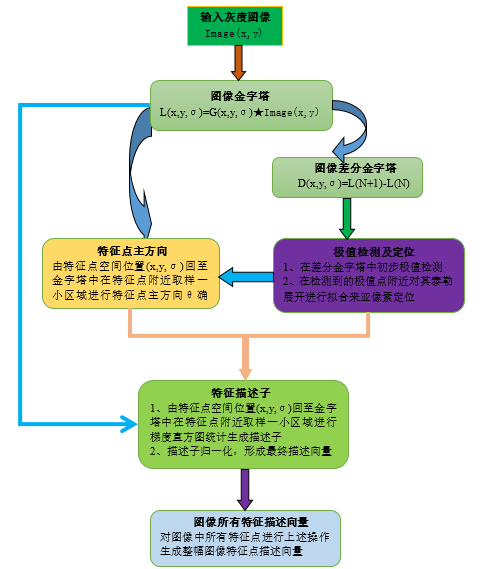二、SIFT算法操作步骤
1、图像金字塔
1.1、高斯金字塔
图像高斯金字塔(Gaussian Pyramid)是采用高斯函数对图像进行模糊以及降采样处理得到。其形成过程可如下图所示：
其中高斯模糊系数计算公式如下：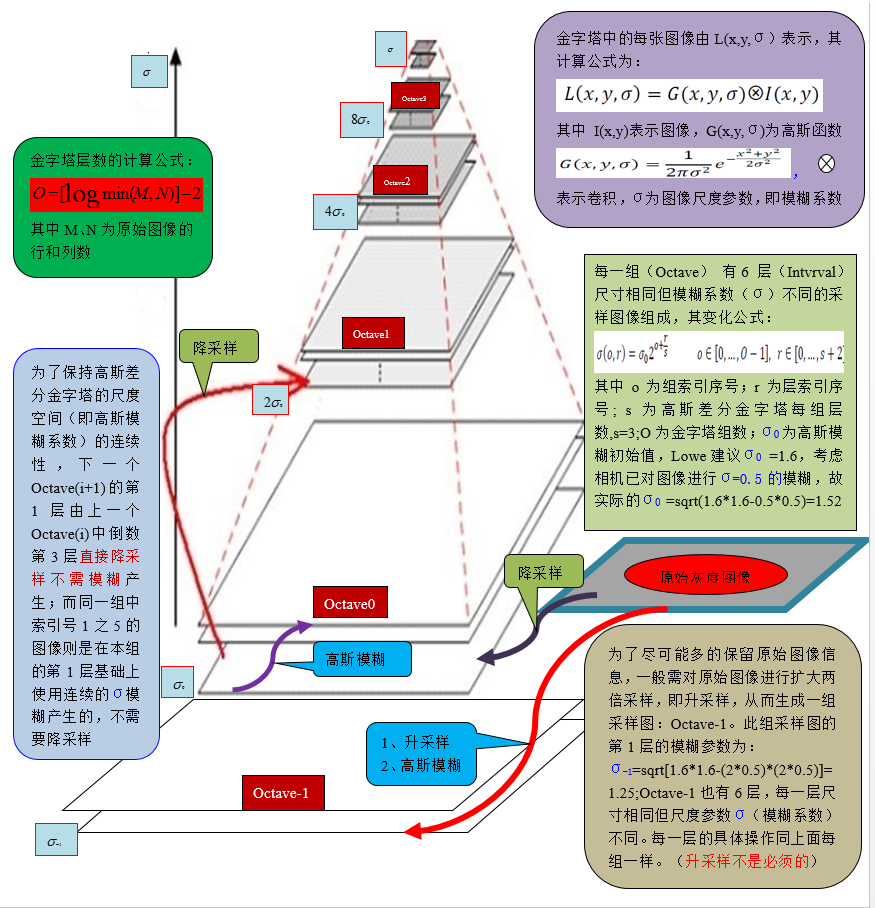其中高斯模糊系数计算公式如下：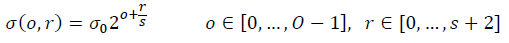1.1.1、高斯函数与图像卷积
根据3σ原则，使用NxN的模板在图像每一个像素点处操作，其中N=[（6σ+1）]且向上取最邻近奇数。
其操作如下图：1.1.2、分离高斯卷积
上面这样直接与图像卷积，速度比较慢，同时图像边缘信息也会损失严重。后来，后来、、、，不知哪位学者发现，可以使用分离的高斯卷积（即先用1xN的模板沿着X方向对图像卷积一次，然后用Nx1的模板沿着Y方向对图像再卷积一次，其中N=[（6σ+1）]且向上取最邻近奇数），这样既省时也减小了直接卷积对图像边缘信息的严重损失。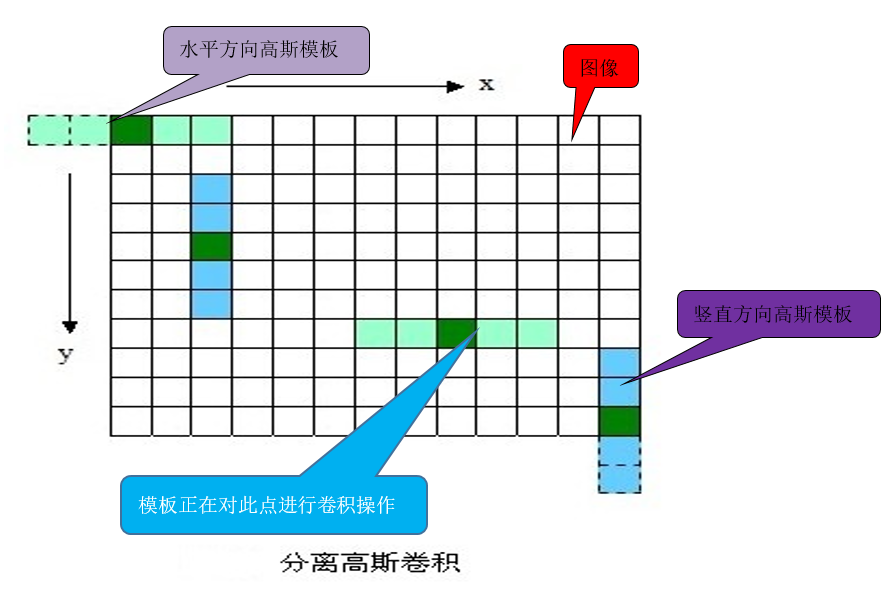1.1.3、高斯金子塔源码分析
for (o = 0; o < octvs; o++)//金字塔组数为octvs，
for (i = 0; i < intvls + 3; i++)//每一组有intvls + 3 层，intvls一般为3
{
if (o == 0 && i == 0)//如果是第一组第1层
gauss_pyr[o][i] = cvCloneImage(base);//base 为原始灰度图像经过升采样或降采样得到的图像
/* base of new octvave is halved image from end of previous octave */
else if (i == 0)//建立非第一组的第1层
gauss_pyr[o][i] = downsample(gauss_pyr[o - 1][intvls]);//降采样图像
/* blur the current octave's last image to create the next one */
else//建立非第一组的非第1层
{
gauss_pyr[o][i] = cvCreateImage(cvGetSize(gauss_pyr[o][i - 1]),IPL_DEPTH_32F, 1);
cvSmooth(gauss_pyr[o][i - 1], gauss_pyr[o][i],CV_GAUSSIAN, 0, 0, sig[i], sig[i]);// sig[i]为模糊系数
}//cvSmooth 为平滑处理函数，也即模糊处理。CV_GAUSSIAN 为选用高斯函数对图像模糊
return gauss_pyr;//返回建好的金字塔

1.2、高斯差分金字塔
2002年Mikolajczyk在详细的实验比较中发现尺度归一化的高斯拉普拉斯函数的极大值和极小值同其它的特征提取函数，例如：梯度，Hessian或Harris角特征比较，能够产生最稳定的图像特征。而Lindeberg早在1994年就发现高斯差分函数（简称DOG算子）与尺度归一化的高斯拉普拉斯函数非常近似。如下式：其中k-1是个常数，并不影响极值点位置的求取。

1.2.1、差分金字塔的建立
差分金字塔的是在高斯金字塔的基础上操作的，其建立过程是：在高斯金子塔中的每组中相邻两层相减（下一层减上一层）就生成高斯差分金字塔.
高斯差分金字塔其操作如下图：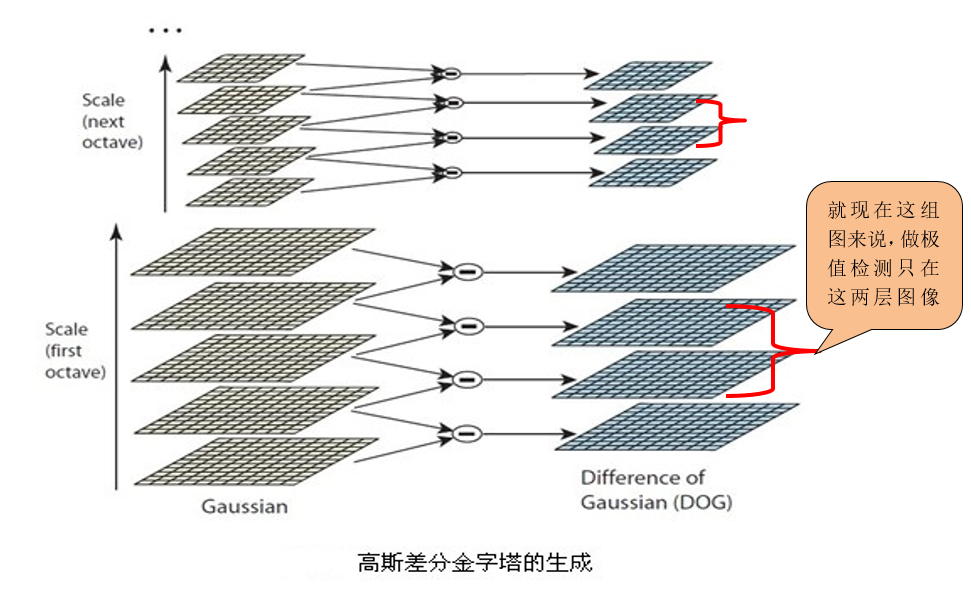1.2.2、差分金字塔源码分析
for (o = 0; o < octvs; o++)//octvs为高斯金字塔组数
for (i = 0; i < intvls + 2; i++)//因为相减，故高斯金字塔中每组有（intvls + 2）层图像
{
dog_pyr[o][i] = cvCreateImage(cvGetSize(gauss_pyr[o][i]),IPL_DEPTH_32F, 1);
cvSub(gauss_pyr[o][i + 1], gauss_pyr[o][i], dog_pyr[o][i], NULL);//cvSub为opencv内置相减函数
}
return dog_pyr;//返回高斯差分金字塔


2、空间极值点（即关键点）检测
关键点是由DOG空间的局部极值点组成的，关键点的初步探查是通过同一组内各DoG相邻两层图像之间比较完成的。为了寻找DoG函数的极值点，每一个像素点要和它所有的相邻点比较，看其是否比它的图像域和尺度域的相邻点大或者小。如图下图所示，中间的检测点和它同尺度的8个相邻点和上下相邻尺度对应的9×2个点共26个点比较，以确保在尺度空间和二维图像空间都检测到极值点。
2.1、极值点检测过程
2.1.1、极值点检测示意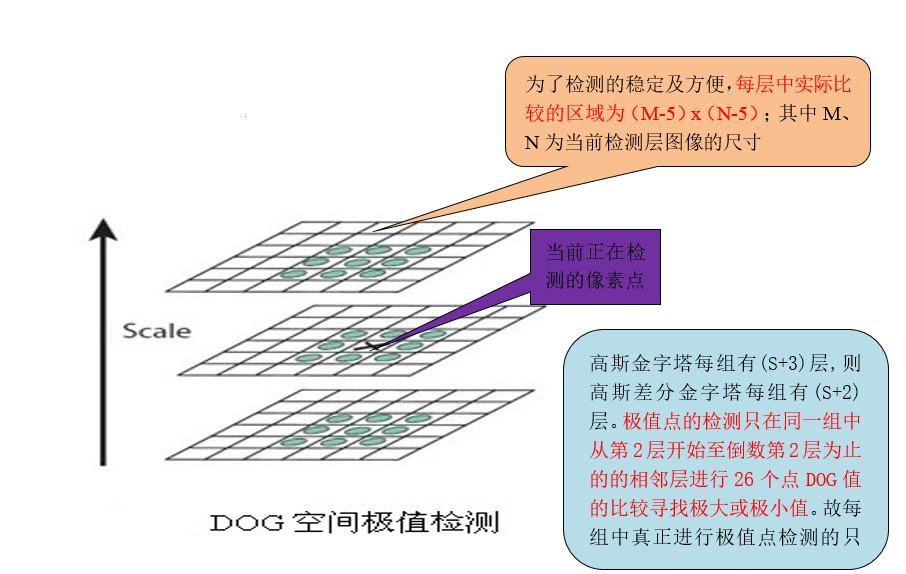2.1.2、极值点检测源码分析
if (val > 0)//极大值检测{
for (i = -1; i <= 1; i++)
for (j = -1; j <= 1; j++)
for (k = -1; k <= 1; k++)
if (val < pixval32f(dog_pyr[octv][intvl + i], r + j, c + k))//pixval32f为提取图像像素位置上的灰度值
return 0;}
else /* check for minimum */
{
for (i = -1; i <= 1; i++)
for (j = -1; j <= 1; j++)
for (k = -1; k <= 1; k++)
if (val > pixval32f(dog_pyr[octv][intvl + i], r + j, c + k))//r c为图像的行数和列数，dog_pyr为高斯差分图
return 0;

2.2、关键点定位
以上方法检测到的极值点是离散空间的极值点，以下通过拟合三维二次函数来精确确定关键点的位置和尺度，同时去除低对比度的关键点和不稳定的边缘响应点(因为DoG算子会产生较强的边缘响应)，以增强匹配稳定性、提高抗噪声能力。
2.2.1、关键点精确定位
离散空间的极值点并不是真正的极值点，下图显示了二维函数离散空间得到的极值点与连续空间极值点的差别。利用已知的离散空间点插值得到的连续空间极值点的方法叫做子像素插值。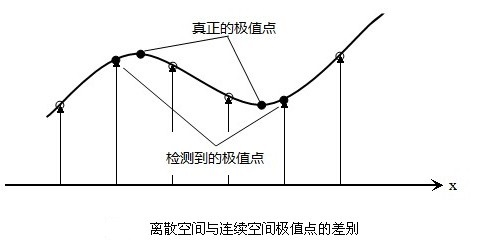为了提高关键点的稳定性，需要对尺度空间DoG函数进行曲线插值。利用DoG函数在尺度空间的Taylor展开式(插值函数)为：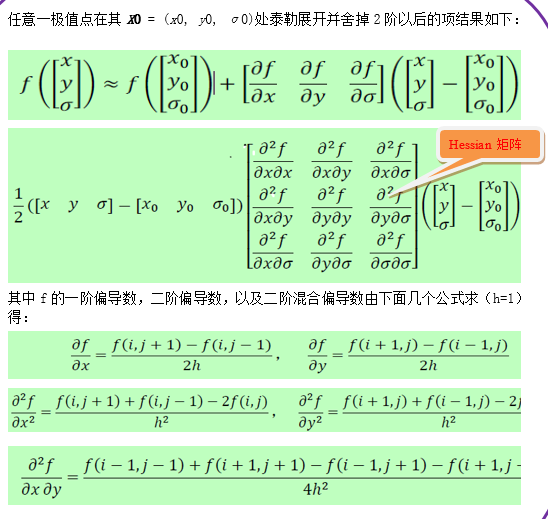上面算式的矩阵表示如下：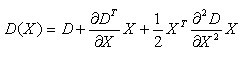其中，X求导并让方程等于零，可以得到极值点的偏移量为：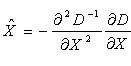对应极值点，方程的值为：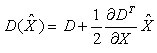其中, X^代表相对插值中心的偏移量，当它在任一维度上的偏移量大于0.5时（即x或y或
σ），意味着插值中心已经偏移到它的邻近点上，所以必须改变当前关键点的位置。同时在新的位置上反复插值直到收敛；也有可能超出所设定的迭代次数或者超出图像边界的范围，此时这样的点应该删除，在Lowe中进行了5次迭代。另外，过小的点易受噪声的干扰而变得不稳定，所以将
小于某个经验值(Lowe论文中使用0.03，Rob Hess等人实现时使用0.04/S)的极值点删除。同时，在此过程中获取特征点的精确位置(原位置加上拟合的偏移量)以及尺度(σ)。

2.2.2、消除边缘响应
一个定义不好的高斯差分算子的极值在横跨边缘的地方有较大的主曲率，而在垂直边缘的方向有较小的主曲率。DOG算子会产生较强的边缘响应，需要剔除不稳定的边缘响应点。获取特征点处的Hessian矩阵，主曲率通过一个2x2 的Hessian矩阵H求出（D的主曲率和H的特征值成正比）：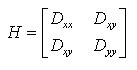假设H的特征值为α和β（α、β代表x和y方向的梯度）且α>β。令α=rβ则有：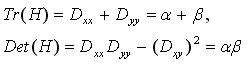其中Tr(H)求取H的对角元素和；Det(H)为求H的行列式值。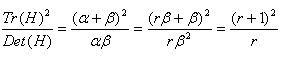则公式(r+1)^2/r的值在两个特征值相等时最小，随着的增大而增大。值越大，说明两个特征值的比值越大，即在某一个方向的梯度值越大，而在另一个方向的梯度值越小，而边缘恰恰就是这种情况。所以为了剔除边缘响应点，需要让该比值小于一定的阈值，因此，为了检测主曲率是否在某域值r下，只需检测: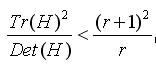论文建议r=10,OpenCv也采用r=10
2.2.3、精确定位中的泰勒插值源码分析
while (i < SIFT_MAX_INTERP_STEPS)//SIFT_MAX_INTERP_STEPS=5为最大迭代次数，避免长时迭代
{
interp_step(dog_pyr, octv, intvl, r, c, &xi, &xr, &xc);// 泰勒展开拟合，xi,xr,xc依次为x、y、σ方向偏移量,
if (ABS(xi) < 0.5  &&  ABS(xr) < 0.5  &&  ABS(xc) < 0.5)//如果当前偏移量绝对值中的每个值均小于0.5，退出迭代
break;
c += cvRound(xc);//计算行坐标，cvRound 为四舍五入。
r += cvRound(xr);
intvl += cvRound(xi);
if (intvl < 1 ||//不在计算的图像层中
intvl > intvls ||//高斯差分每组的层数为intvls
c < SIFT_IMG_BORDER ||//靠近图像边缘5个像素的区域不做检测，SIFT_IMG_BORDER=5,
r < SIFT_IMG_BORDER ||
c >= dog_pyr[octv]->width - SIFT_IMG_BORDER ||//靠近图像边缘5个像素的区域不做检测
r >= dog_pyr[octv]->height - SIFT_IMG_BORDER)
{
return NULL;
}
i++;//迭代计数
}
static void interp_step(IplImage*** dog_pyr, int octv, int intvl, int r, int c,double* xi, double* xr, double* xc)
{
CvMat* dD, *H, *H_inv, X;
double x = { 0 };
dD = deriv_3D(dog_pyr, octv, intvl, r, c);//一阶偏导数
H = hessian_3D(dog_pyr, octv, intvl, r, c);//Hessian 矩阵即二阶导数组成的矩阵
H_inv = cvCreateMat(3, 3, CV_64FC1);
cvInvert(H, H_inv, CV_SVD);//求Hessian矩阵的逆矩阵
cvInitMatHeader(&X, 3, 1, CV_64FC1, x, CV_AUTOSTEP);
cvGEMM(H_inv, dD, -1, NULL, 0, &X, 0); //cvGEMM为矩阵乘法，//第一个矩阵的系数；//H_inv、dD第一二个矩阵//-1矩阵前的常数//X结果矩阵
cvReleaseMat(&dD);
cvReleaseMat(&H);
cvReleaseMat(&H_inv);
*xi = x;
*xr = x;
*xc = x;
}

3、关键点方向分配
为了使描述符具有旋转不变性，需要利用图像的局部特征为给每一个关键点分配一个基准方向。使用图像梯度的方法求取局部结构的稳定方向。
3.1、特征点的梯度
3.1.1、梯度的计算
对于在DOG金字塔中检测出的关键点点，采集其所在高斯金字塔图像3σ领域窗口内像素的梯度和方向分布特征。梯度的模值和方向如下：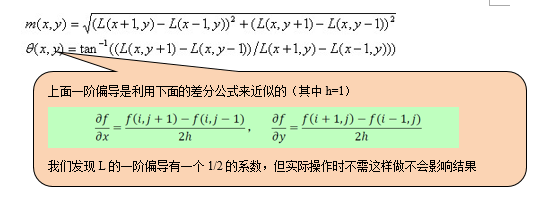L为关键点所在的尺度空间值，按Lowe的建议，梯度的模值m(x,y)按 σ=1.5σ_oct 的高斯分布加成，按尺度采样的3σ原则，领域窗口半径为 3x1.5σ_oct。
3.1.1、梯度直方图
在完成关键点的梯度计算后，使用直方图统计领域内像素的梯度和方向。梯度直方图将0~360度的方向范围分为36个柱(bins)，其中每柱10度。如图5.1所示，直方图的峰值方向代表了关键点的主方向，(为简化，图中只画了八个方向的直方图)。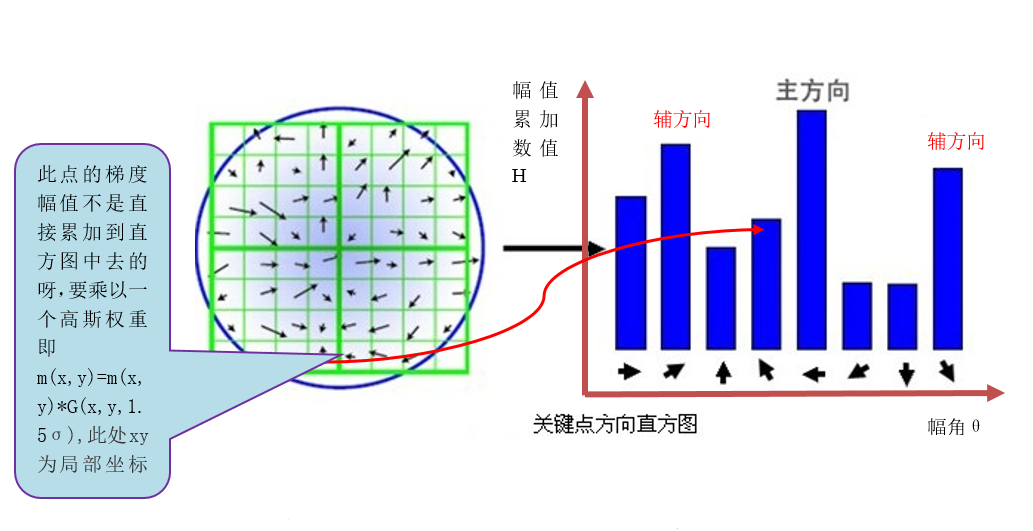3.2、特征点主方向的确定
方向直方图的峰值则代表了该特征点处邻域梯度的方向，以直方图中最大值作为该关键点的主方向。为了增强匹配的鲁棒性，只保留峰值大于主方向峰值80％的方向作为该关键点的辅方向。因此，对于同一梯度值的多个峰值的关键点位置，在相同位置和尺度将会有多个关键点被创建但方向不同。仅有15％的关键点被赋予多个方向，但可以明显的提高关键点匹配的稳定性。实际编程实现中，就是把该关键点复制成多份关键点，并将方向值分别赋给这些复制后的关键点，并且，离散的梯度方向直方图要进行插值拟合处理，来求得更精确的方向角度值。
3.2.1、梯度图像的平滑处理
为了防止某个梯度方向角度因受到噪声的干扰而突变，我们还需要对梯度方向直方图进行平滑处理。Opencv  所使用的平滑公式为：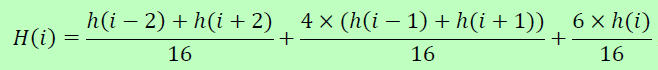其中i∈[0,35],h 和H 分别表示平滑前和平滑后的直方图。由于角度是循环的，即00=3600，如果出现h(j)，j超出了(0,…,35)的范围，那么可以通过圆周循环的方法找到它所对应的、在00=3600之间的值，如h(-1)
= h(35)。
3.2.2、梯度直方图抛物线插值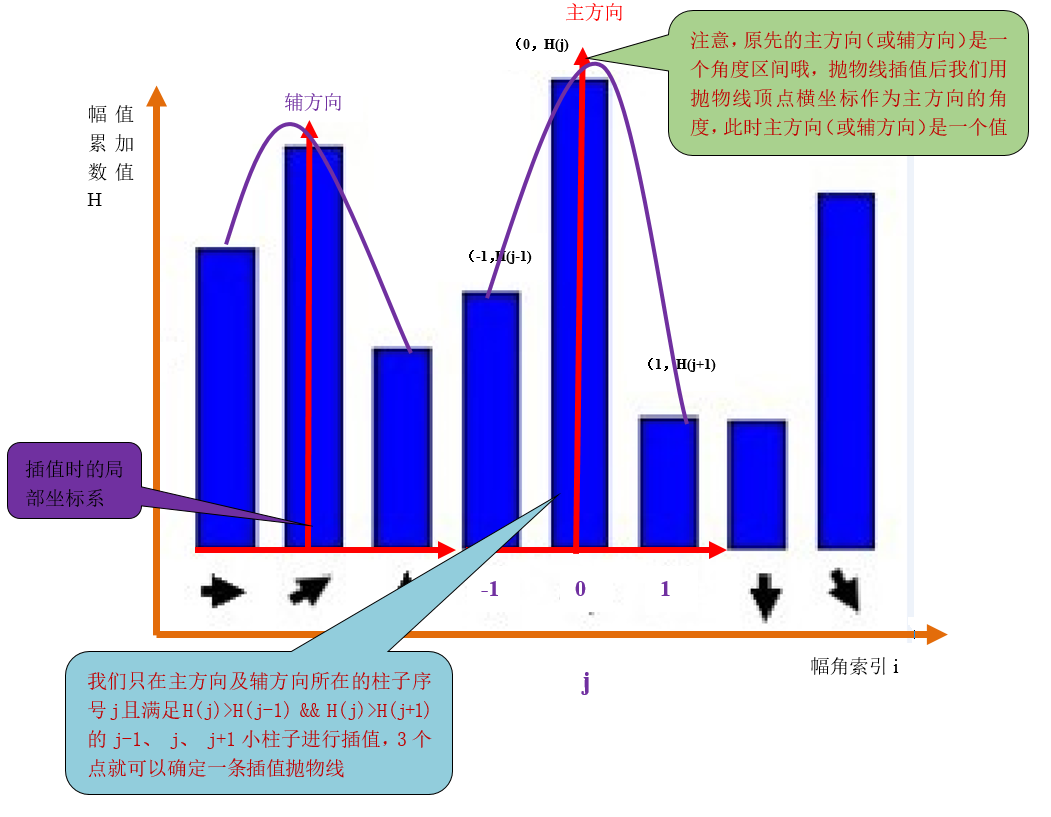假设我们在第i个小柱子要找一个精确的方向，那么由上面分析知道：
设插值抛物线方程为h(t)=at2+bt+c,其中a、b、c为抛物线的系数，t为自变量，t∈[-1,1],此抛物线求导并令它等于0。
即h(t)´=0
得tmax=-b/(2a)
现在把这三个插值点带入方程可得：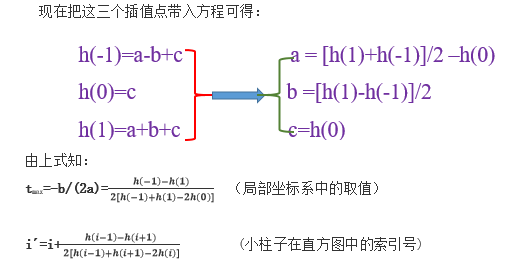3.2.3、抛物线插值源码分析
#define interp_hist_peak( l, c, r ) ( 0.5 * ((l)-(r)) / ((l) - 2.0*(c) + (r)) )//插值计算式，l为左侧柱子值，r为左侧柱子值
static void add_good_ori_features(CvSeq* features, double* hist, int n,
double mag_thr, struct feature* feat)//精确主方向及辅方向
{
struct feature* new_feat;
double bin, PI2 = CV_PI * 2.0;//CV_PI=pi
int l, r, i;
for (i = 0; i < n; i++)// 直方图有n=36个小柱子
{
l = (i == 0) ? n - 1 : i - 1;//把小柱子看成是循环的，角度的取值为0-360即一个圆周
r = (i + 1) % n;
//只对小柱子的值大于等于主峰80%且此小柱子比左右两边小柱子都高的柱子进行抛物线插值
if (hist[i] > hist[l] && hist[i] > hist[r] && hist[i] >= mag_thr)// mag_thr为>=80%的最高峰值
{
bin = i + interp_hist_peak(hist[l], hist[i], hist[r]);//interp_hist_peak 插值函数
bin = (bin < 0) ? n + bin : (bin >= n) ? bin - n : bin;//角度取值约束在0-360之间，且是连续循环的
new_feat = clone_feature(feat);//幅值特征点
new_feat->ori = ((PI2 * bin) / n) - CV_PI;//？
cvSeqPush(features, new_feat);
free(new_feat);
}

至此，图像的关键点已检测完毕，每个关键点有三个信息：位置、所处尺度、方向。由此可以确定一个SIFT特征区域。
4、特征点描述符
通过以上步骤，对于每一个关键点，拥有三个信息：位置、尺度以及方向。接下来就是为每个关键点建立一个描述符，使其不随各种变化而改变，比如光照变化、视角变化等等。并且描述符应该有较高的独特性，以便于提高特征点正确匹配的概率。
4.1、特征的生成过程4.1.1、确定计算描述子所需的区域
将关键点附近的区域划分为d*d(Lowe建议d=4)个子区域，每个子区域作为一个种子点，每个种子点有8个方向。考虑到实际计算时，需要采用三线性插值，所需图像窗口边长为3x3xσ_oct
x(d+1)  。在考虑到旋转因素(方便下一步将坐标轴旋转到关键点的方向)，如下图6.1所示，实际计算所需的图像区域半径为：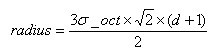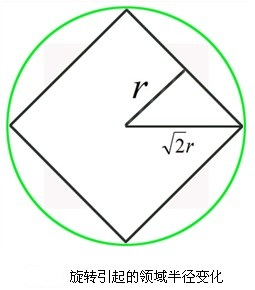4.1.2、坐标轴旋转至主方向
将坐标轴旋转为关键点的方向，以确保旋转不变性。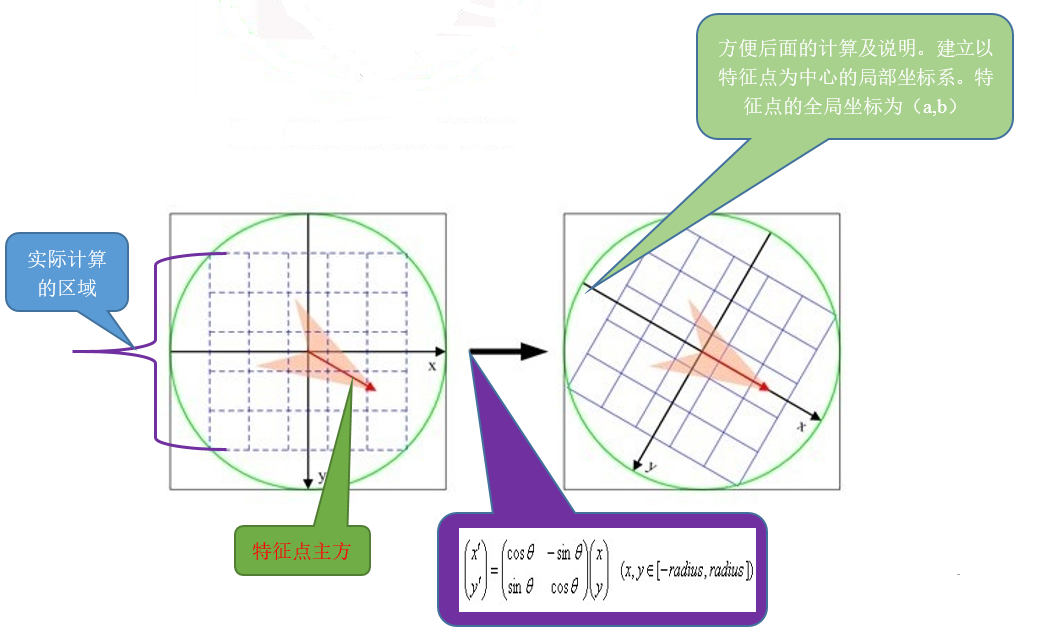4.1.3、梯度直方图的生成
将邻域内的采样点分配到对应的子区域内，将子区域内的梯度值分配到8个方向上，计算其权值。
旋转后的采样点
落在子区域的下标为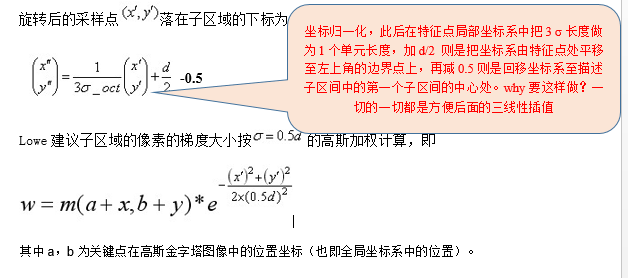4.1.4、三线性插值
插值计算每个种子点八个方向的梯度。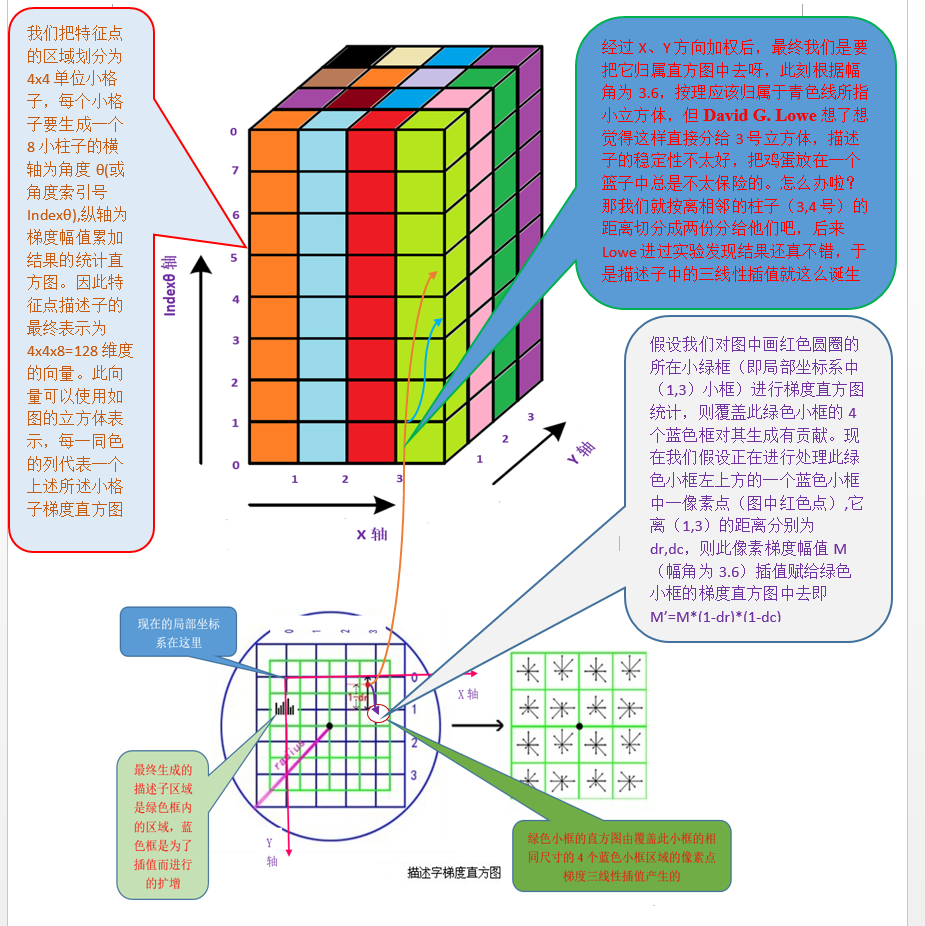采样点在子区域中的下标(x'',y'')                              (图中蓝色窗口内红色点)线性插值，计算其对每个种子点的贡献。如图中的红色点，落在第0行和第1行之间，对这两行都有贡献。对第0行第3列种子点的贡献因子为dr，对第1行第3列的贡献因子为1-dr，同理，对邻近两列的贡献因子为dc和1-dc，对邻近两个方向的贡献因子为do和1-do。则最终累加在每个方向上的梯度大小为：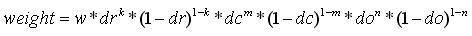其中k，m，n为0（像素点超出了对要插值区间的四个邻近子区间所在范围）或为1（像素点处在对要插值区间的四个邻近子区间之一所在范围）。
4.1.5、特征描述子
如上统计的4*4*8=128个梯度信息即为该关键点的特征向量。
特征向量形成后，为了去除光照变化的影响，需要对它们进行归一化处理，对于图像灰度值整体漂移，图像各点的梯度是邻域像素相减得到，所以也能去除。得到的描述子向量为H=(h1,h2,.......,h128)，归一化后的特征向量为L=(L1,L2,......,L128)，则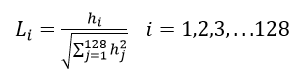4.1.6、描述子的门限化
非线性光照，相机饱和度变化对造成某些方向的梯度值过大，而对方向的影响微弱。因此设置门限值(向量归一化后，一般取0.2)截断较大的梯度值(大于0.2的则就令它等于0.2，小于0.2的则保持不变)。然后再进行一次归一化处理，提高特征的鉴别性。
4.2、描述子相关分析
用一组图来概括描述子的生成过程
4.2.1、描述子生成总括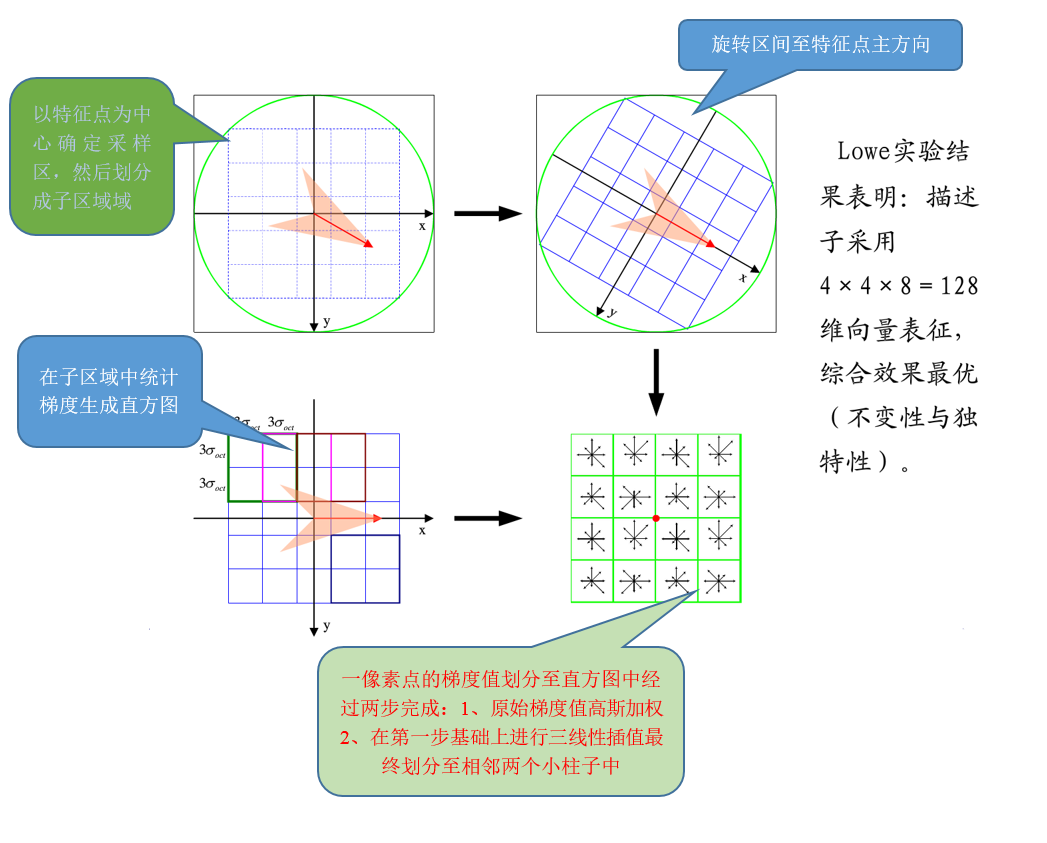4.2.3、描述子三线性插值源码分析
static void interp_hist_entry(double*** hist, double rbin, double cbin,
double obin, double mag, int d, int n)
{
double d_r, d_c, d_o, v_r, v_c, v_o;
double** row, *h;
int r0, c0, o0, rb, cb, ob, r, c, o;
r0 = cvFloor(rbin);//向下取整
c0 = cvFloor(cbin);
o0 = cvFloor(obin);
d_r = rbin - r0;//小数余项
d_c = cbin - c0;
d_o = obin - o0;
for (r = 0; r <= 1; r++)//沿着行方向不超过1个单位长度
{
rb = r0 + r;
if (rb >= 0 && rb < d)//如果此刻还在真正的描述子区间内
{
v_r = mag * ((r == 0) ? 1.0 - d_r : d_r);//d_r = rbin - r0;
row = hist[rb];
for (c = 0; c <= 1; c++)//沿着行方向不超过1个单位长度
{
cb = c0 + c;
if (cb >= 0 && cb < d)
{
v_c = v_r * ((c == 0) ? 1.0 - d_c : d_c);
h = row[cb];
for (o = 0; o <= 1; o++)//沿着直方图方向不超过1个单位角度
{
ob = (o0 + o) % n；//n=8，8个小柱子
v_o = v_c * ((o == 0) ? 1.0 - d_o : d_o);
h[ob] += v_o;
}
}
}
}

通过上面的1至4个大步骤就可以完成SIFT算法对图像特征点的提取。至此SIFT算法完结。图像特征提取是图像匹配的基础，经过此算法提取出来的特征点用于后续的图像特征匹配和特征识别中，关于图像特征匹配相关内容将在后续讲解。

参考文献
1、sift算法详解及应用（课件）。（本文档简明扼要的简述了SIFT算法和图像匹配以及匹配修正。图文并茂，一览全貌）
http://wenku.baidu.com/view/87270d2c2af90242a895e52e.html?re=view
2、SIFT算法详解（sift操作过程理论通俗，尤其是高阶泰勒展开式及高阶导数分析的很好，对理解亚像素定位拟合中的图像具体编程操作很有用）
http://blog.csdn.net/zddblog/article/details/7521424
3、SIFT特征分析与源码解读（1模拟金字塔的过程解释的很详细，带有动画模拟；2 在寻找特征点进行亚像素定位拟合中的图像很形象）

http://blog.csdn.net/xw20084898/article/details/16832755
4、【OpenCV】SIFT原理与源码分析：关键点描述（对关键点描述子区域的取舍讲解的很详细）

http://blog.csdn.net/xiaowei_cqu/article/details/8113565
5、【OpenCV】SIFT原理与源码分析（对sift 算法采用分部分叙述且带有源码分析说明）

http://blog.csdn.net/xiaowei_cqu/article/details/8069548
6、opencv2.4.9sift源码分析(1赵春江的这篇文章是我目前看到分析sift算法比较全面的；2尤其给出了使用三维直方图来分析三线性插值，对理解描述子的生成作用很大；3 给出了源码分析和演示结果）
http://wenku.baidu.com/view/d7edd2464b73f242336c5ffa.html
7、九之再续：教你一步一步用c语言实现sift算法、下
（1算法中寻找主方向使用的抛物线插值拟合方法；2 描述子三次插值）

http://blog.csdn.net/v_JULY_v/article/details/6246213
8、RobHess的SIFT源码分析：综述(各个子程序详解及分析很细致，一概全貌）

http://blog.csdn.net/masibuaa/article/details/9191309
9、特征点检测学习_1(sift算法)（1这篇文章没有太多理论分析，但结合QT和OpenCV做出了生动的sift算法匹配演示，有图很直观生动呀，用程序配图一目了然；2
简述对robhess 的c版本sift代码在c++中的使用注意问题 ）

10、OpenCV 中c版本sift源代码网址
http://blogs.oregonstate.edu/hess/code/sift/
11、【特征匹配】SIFT原理与C源码剖析（这个也不错，图文并茂，还带有
源码分析，总体来说是以程序带动问题分析）

http://blog.csdn.net/luoshixian099/article/details/47377611
12、插值与拟合（对多项式及其插值讲解还不错）
13、线性插值与抛物线插值（对这两种插值讲解的很详细，是目前发现最 好的一版
http://www.docin.com/p-711275966.html
14、奇异值分解（对奇异值怎么来的讲解比较细致）
http://blog.sina.com.cn/s/blog_53eb0fdf0101sfu1.html


展开全文•opencv 立体视觉 c++
• ## 特征点提取opencv

千次阅读 2019-04-19 23:05:26
opencv中提供了Harris角检测的接口，即cv::cornerHarris() 缺陷：角是像素级别的，速度较慢 dst = cv2.cornerHarris(gray,2,3,0.04) img - 数据类型为 float32 的输入图像 blockSize - 角检测中要考虑的领域...
• before：本篇博文先主要叙述ORB特征点提取算法，包括对其分解， FAST特征点的生成，BRIEF描述子的生成。然后对FPGA实现特征点 提取进行简介。铺垫好这些基础后，在下一篇博文中，仔细介绍如何 采用FPGA来实现高速的...实时图像处理 FPGA ORB算子
• 转自：https://blog.csdn.net/dcrmg/article/details/52577555Sift之前的江湖在Sift横空出世之前，特征点检测与匹配江湖上占据霸主地位的是角点检测家族。先来探究一下角点家族不为人知的恩怨情仇。角点家族的族长是...
• ## SIFI特征点提取

千次阅读 2017-03-23 22:58:11
尺度不变特征变换匹配算法详解 Scale Invariant Feature Transform(SIFT) Just For Fun zdd zddmail@gmail.com 对于初学者，从David G.Lowe的论文到实现，有许多鸿沟，本文帮你跨越。 1、SIFT...C++
• 1、sift特征原理的描述 1.1sift概述： 首先我了解到了兴趣点的...而描述子就是对兴趣点提取特征的过程。sift是一种特征描述子。该描述子具有尺度不变性和光照不变性。 1.2sift特征检测的步骤： sift特征检测...
• 过年期间闲来无事，就想来把这半年学习的一些视觉知识（视觉slam相关）做个梳理，就以这篇图像特征点提取与匹配作为开头吧。 一、关键点与描述子 关键点：图像上某些特殊的、具有代表的点（常见表示就是图像上的...
• opencv 特征点提取、匹配（一） opencv中特征点提取和匹配步骤： 提取特征点 生成特征点的描述子 特征点匹配 opencv对应类： 图像特征点的提取 — FeatureDetector 特征点描述子生成 – DescriptorExtractor ...opencv 匹配
• 1. 特征点提取的意义 2.角点 3. Harris角点检测的基本原理 4.Harris角点检测算法的步骤 5.Harris角点提取算法设计 function [ptx,pty] = ...
• 1. FAST（featuresfrom accelerated segment test）算法 ... 特征点检测和匹配是计算机视觉中一个很有用的技术。在物体检测，视觉跟踪，三维常年...很多传统的算法都很耗时，而且特征点检测算法只是很多复杂图像处理里中opencv 计算机视觉 图片
• ## 特征点提取与匹配

千次阅读 2017-10-29 15:15:24
兴趣点（interest points），或称作关键点（keypoints）、特征点（feature points） 被大量用于解决物体识别，图像识别、图像匹配、视觉跟踪、三维重建等一系列的问题。不再观察整幅图，而是选择某些特殊的点，然后...opencv
• ## 指纹识别源代码（2）-特征点提取

万次阅读 多人点赞 2019-11-26 19:14:35
上图为上一步拿到的图像预处理之后的细化图。在这个基础之上我们进行下一步操作。...指纹特征提取的主要目的是计算指纹核心(Core)和细节(Minutia)的特征信息。在提取指纹核心时，采用的是Poi...
• 接下来，我们开始进入正题，特征点提取与匹配的实践操作。本篇文章中，我将着重介绍SIFT这种特征点以及做一个简单的特征点提取实践。 1.什么是特征点？ 首先我们来介绍一下什么是特征点，从字面的意思上来解释就是...
• 将现有的ASM公开算法包进行对比，且以ASMLibrary为例，进行了简单实验，保存人脸68个特征点坐标。另外也罗列了深度学习在人脸特征点检测的相关英文教程，并进行了简单总结。Theano Caffe
• 图像配准 本文为原创文章，转载请注明...匹配：指寻找两幅影像中相似的部分(基于特征点或灰度等)，从而找到与搜索图像相似的图像 配准：将不同时间、不同传感器(成像设备)或不同条件下(天候、照度、摄像位置和角度...ORB
• ## 一起学ORBSLAM（2）ORB特征点提取

万次阅读 多人点赞 2018-06-05 22:27:57
ORBSLAM2的主要特点在于他的所有工程实现都用到了ORB这个特征点提取方法，包括他实现的在线单目，双目以RGBD的SLAM,下面我们就看看他是怎么一步一步一步一步一步一步一步一步一步一步实现ORB特征的提取的。...
...